# Restricted Topological Products

In this section we describe a topological tool, which we need in order to define adeles (see Definition 20.3.1).

Definition 20.2.1 (Restricted Topological Products)   Let, for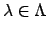, be a family of topological spaces, and for almost all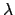let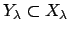be an open subset of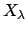. Consider the space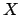whose elements are sequences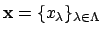, where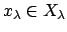for every, and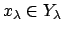for almost all. We givea topology by taking as a basis of open sets the sets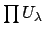, where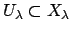is open for all, and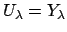for almost all. We callwith this topology the of thewith respect to the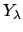.

Corollary 20.2.2   Let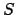be a finite subset of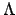, and let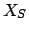be the set of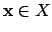withfor all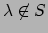, i.e.,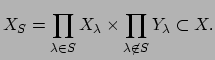Thenis an open subset of, and the topology induced onas a subset ofis the same as the product topology.

The restricted topological product depends on the totality of the, but not on the individual:

Lemma 20.2.3   Let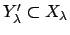be open subsets, and suppose that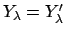for almost all. Then the restricted topological product of thewith respect to the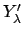is canonically isomorphic to the restricted topological product with respect to the.

Lemma 20.2.4   Suppose that theare locally compact and that theare compact. Then the restricted topological productof theis locally compact.

Proof. For any finite subsetof, the open subset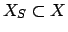is locally compact, because by Lemma 20.2.2 it is a product of finitely many locally compact sets with an infinite product of compact sets. (Here we are using Tychonoff's theorem from topology, which asserts that an arbitrary product of compact topological spaces is compact (see Munkres's Topology, a first course, chapter 5).) Since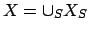, and theare open in, the result follows.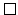The following measure will be extremely important in deducing topological properties of the ideles, which will be used in proving finiteness of class groups. See, e.g., the proof of Lemma 20.4.1, which is a key input to the proof of strong approximation (Theorem 20.4.4).

Definition 20.2.5 (Product Measure)   For all, suppose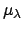is a measure onwith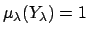whenis defined. We define the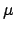onto be that for which a basis of measurable sets is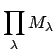where each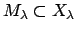has finite-measure and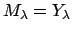for almost all, and where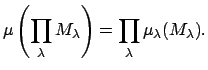William Stein 2004-05-06